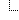﻿QuantumAlgorithms Class
Cove - A Practical Quantum Computer Programming Framework
QuantumAlgorithms Class
NamespacesCove.LocalSimulationQuantumAlgorithms
This class provides functions to perform common quantum algorithms. This allows users to perform some typical quantum algorithms without being concerned about the implementation details.Declaration Syntax
 C# Visual Basic Visual C++
```public class QuantumAlgorithms : IQuantumAlgorithms,
ICoveObject```
```Public Class QuantumAlgorithms _
Implements IQuantumAlgorithms, ICoveObject```
```public ref class QuantumAlgorithms : IQuantumAlgorithms,
ICoveObject```Members
 All Members Constructors Methods PublicProtected InstanceStatic DeclaredInherited
IconMemberDescriptionQuantumAlgorithms()()()AddN(array<Int32>[]()[], array<Int32>[]()[], array<Int32>[]()[])
Return the operations needed to perform Add over two registers of equal size.AddN(array<Int32>[]()[], array<Int32>[]()[], array<Int32>[]()[], array<Int32>[]()[]%)
Return the operations needed to perform Add over two registers of equal size.AddNInverse(array<Int32>[]()[], array<Int32>[]()[], array<Int32>[]()[])
Return the operations needed to perform Add Inverse over two registers of equal size. Add inverse is subtraction.AddNInverse(array<Int32>[]()[], array<Int32>[]()[], array<Int32>[]()[], array<Int32>[]()[]%, Int32%)
Return the operations needed to perform Add Inverse over two registers of equal size. Add inverse is subtraction.Carry(Int32, Int32, Int32, Int32)
Return the operations to perform the carry gate.CarryInverse(Int32, Int32, Int32, Int32)
Return the operations to perform the inverse carry.ControlledReset(Int32, array<Int32>[]()[], ClassicalResult)
The controlled reset operation. If the control qubit is 1 then all the target indexes are reset to 0.Equals(Object)
Determines whether the specified Object is equal to the current Object.
(Inherited from Object.)Factor(Int32, Int32%, Int32%)
Factor a number using Shor's algorithm.FactoringUf(array<Int32>[]()[], array<Int32>[]()[], Int32)
Perform Uf for factoringFinalize()()()
Allows an Object to attempt to free resources and perform other cleanup operations before the Object is reclaimed by garbage collection.
(Inherited from Object.)GetHashCode()()()
Serves as a hash function for a particular type. GetHashCode()()() is suitable for use in hashing algorithms and data structures like a hash table.
(Inherited from Object.)GetRegisterEPRPair()()()
Get the EPR Pair (Phi plus, or Einstein Podolsky Rosen), which is defined as the 2 qubit register: (1 / sqrt(2))(|00> + |11>) This is also a bell state.GetRegisterGHZ()()()
Get the GHZ (Greenberger-Horne-Zeilinger) register, which is defined as the three qubits in the state: (1 / sqrt(2))(|000> + |111>)GetRegisterPhiMinus()()()
Get the Phi minus register, which is defined as the two qubits: (1 / sqrt(2))(|00> - |11>)j This is also a bell state.GetRegisterPhiPlus()()()
Get the EPR Pair (Phi plus, or Einstein Podolsky Rosen), which is defined as the 2 qubit register: (1 / sqrt(2))(|00> + |11>) This is also a bell state.GetRegisterPsiMinus()()()
Get the Psi minus register, which is defined as the two qubits: (1 / sqrt(2))(|01> - |10>) This is also a bell state.GetRegisterPsiPlus()()()
Get the Psi plus register, which is defined as the two qubits: (1 / sqrt(2))(|01> + |10>) This is also a bell state.GetRegisterW()()()
Get the W register, which is defined as the three qubits in the state: (1 / sqrt(3))(|100> + |010> + |001>)GetType()()()
Gets the Type of the current instance.
(Inherited from Object.)MemberwiseClone()()()
Creates a shallow copy of the current Object.
(Inherited from Object.)ModularNAdder(array<Int32>[]()[], array<Int32>[]()[], array<Int32>[]()[], array<Int32>[]()[])
Performs modular addition of X and Y: (x + y) mod n. All index arrays must be of equal length.NegatedCNot(Int32, Int32)
Returns the operations to perform the negated CNot. The normal CNot operation flips the target qubit when the control is 1, otherwise no change is made. This (the negated) flips the target when the control is 0 instead of 1.QuantumFourierTransform(array<Int32>[]()[])
Get the operations to apply the Quantum Fourier Transform.QuantumFourierTransformInverse(array<Int32>[]()[])
Get the operations to apply the inverse Quantum Fourier Transform.Sum(Int32, Int32, Int32)
Return the operations that perform Sum.SwapIndexes(array<Int32>[]()[], array<Int32>[]()[])
Swap the first indexes and the second indexes. Each qubit in element x of FirstSwapIndexes will be swapped with element x of SecondSwapIndexes. Example: A register is ordered 0, 1, 2, 3. If FirstIndexes = 0, 2 and SecondIndexes = 3, 1 then the resulting order will be 3, 1, 2, 0.ToString()()()
Returns a String that represents the current Object.
(Inherited from Object.)Inheritance Hierarchy
 ObjectQuantumAlgorithms

Assembly: Cove.LocalSimulation (Module: Cove.LocalSimulation) Version: 1.0.0.0 (1.0.0.0)